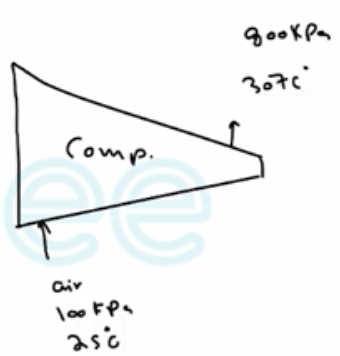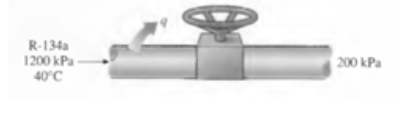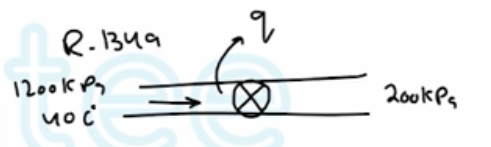Need Help?

Subscribe to Thermodynamics

###### \${selected_topic_name}
• Notes

$\begin{array}{l}{\text { Nitrogen is compressed by an adiabatic compressor from } 100 \mathrm{kPa} \text { and } 25^{\circ} \mathrm{C} \text { to } 800 \mathrm{kPa} \text { and } 307^{\circ} \mathrm{C} \text { . }} \\ {\text { Calculate the entropy generation for this process, in } \mathrm{kJ} / \mathrm{kg}-\mathrm{K} \text { . }}\end{array}$$A-2 a \& A-2 b \rightarrow C p=1.048$

$R=0.2968$

$\dot{S}_{i n}-\dot{S}_{out}+S_{gen}=\Delta \dot S_{sys}$

$\dot m_{1} s_{1}-\dot{m}_{2} s_{2}+\dot{S}_{gen}=0$

$\dot{S}_{gen}=\dot{m}\left(S_{2}-S_{1}\right)$

$=C_p \ln \frac{T_2}{T_1}-R \ln \frac{P_2}{P_{1}}$

$=1.048 \ln \frac{307+273}{25+273}-0.2968 \ln \frac{800kPa}{100kPa}$

$=0.0807 kJ / k g \cdot k$

$\begin{array}{l}{\text { Refrigerant-134a is throttled from } 1200 \mathrm{kPa} \text { and } 40^{\circ} \mathrm{C} \text { to } 200 \mathrm{kPa} \text { . Heat is lost from the refrigerant }} \\ {\text { in the amount of } 0.5 \mathrm{kJ} / \mathrm{kg} \text { to the surroundings at } 25^{\circ} \mathrm{C} \text { Determine }(, \mathrm{a}) \text { the exit temperature of }} \\ {\text { the refrigerant and (b) the entropy generation during this process. }}\end{array}$$\left.\begin{array}{l}{P_{1}=1200 \mathrm{kPa}} \\ {T_{1}=40c^{\circ}}\end{array}\right]\rightarrow A-13 \$$\begin{array}{l}{h_{1}=108 \cdot 23 \mathrm{kJ} / \mathrm{kg}} \\ {S_{1}=0.39424 \mathrm{KJ/kg}}\end{array}$

$q_{\text {out }}=h_{1}-h_{2}$

$h_{2}=h_{1}-q_{\text {out }}$

$=108.23-0.5=107.73 \mathrm{kJ/kg}$

$\left.\begin{array}{l}{P_{2}=200 \mathrm{kPa}} \\ {h_{2}=10{7.73}}\end{array}\right\} \longrightarrow$$\begin{array}{l}{T_{2}=10.09 c^{\circ}} \\ {S_{2}=0.418 kJ/kg.k}\end{array}$

$\Delta S_{ R .134a}=S_2-S_1=0.418-0.39424=0.02375$

$\Delta S_{surr}=\frac{q_{\text {out }}}{T}=\frac{0.5}{25+273}=0.001678$

$S _{ge n}=\Delta S_{\text {total }}=\Delta S_R+\Delta S_{surr}=0.02543 kJ/kg.k$